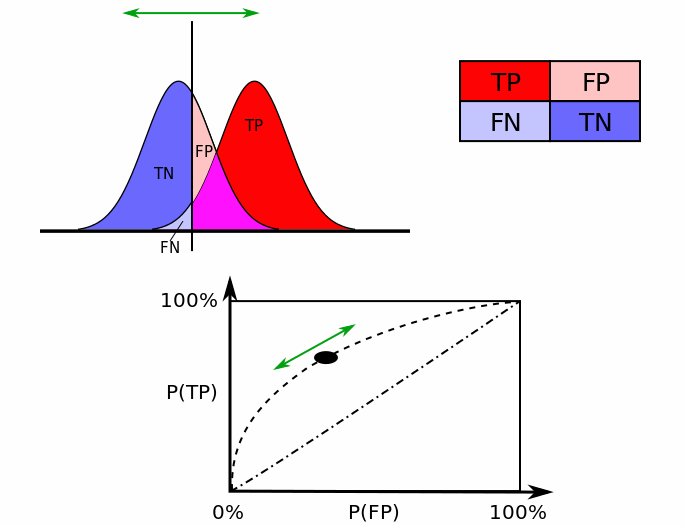#### 怎么直观理解ROC AUC的概率统计意义？

统计/机器学习 监督式学习 模型验证 开放问题    浏览次数：11909        分享

2

ROC AUC是指ROC曲线下面的面积，越接近于1越好，但是ROC AUC有什么更深层、直观的概率统计的意义吗？杨业勇   2017-09-28 13:07

##### 3个回答
7

ROC AUC有个非常直白的概率意义。

ROC AUC = 0.8的意思是说，随机挑选个标签为1的样本，它被排在随机的0样本的前面的概率是0.8。显然ROC AUC是0.5的话，就说明这个模型和随便猜没什么两样。

SofaSofa数据科学社区DS面试题库 DS面经五道口少年   2017-09-29 11:45

4

ROC就是把$T$从$\infty$到$-\infty$，所有$P(FP),P(TP)$的点连成的曲线。

$P(FP)=FPR(T)=\int_{T}^{\infty} f_0(x) dx$

$P(TP)=TPR(T)=\int_{T}^{\infty} f_1(x) dx$$AUC=\int_{\infty}^{-\infty}P(TP)P'(FP)dT$

$AUC=\int_{\infty}^{-\infty}\int_{T}^{\infty} f_1(x) dx (-f_0(T)) dT$

$=\int_{-\infty}^{\infty}\int_{T}^{\infty} f_1(x) dx f_0(T) dT$

$=\int_{-\infty}^{\infty}\int_{x_0}^{\infty} f_1(x_1) f_0(x_0) dx_1dx_0$

$=P(x_1>x_0|x_1 \sim f_1,x_0 \sim f_0)$

SofaSofa数据科学社区DS面试题库 DS面经Zealing   2018-08-23 00:53

1

• AUC==1: 模型可以100%准确分类
• AUC==0.5: 效果等于随机
• AUC==0: 模型分类100%错误
SofaSofa数据科学社区DS面试题库 DS面经holidy   2018-08-21 02:20相关主题